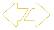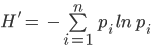#### Tree Species Diversity

Latest Score:

4.1/5

in 2019

Weight: 8%score trend is flat over timeTree species diversity represents both the number of different tree species and the relative abundance of each species in the forest.  As tree species diversity increases so does the forest’s capacity to support other biodiversity in the form of birds, animals, insects, and fungi. Diverse forests are more resilient to stress, recover more quickly following damage, and can be more productive1. Here, forest diversity is assessed using the Shannon-Weiner Index using the proportion of trees per species. A higher score means that the forest has a high number of species with a fairly equal abundance of each of those species.

1Whittaker, R.H., 1972. Evolution and measurement of species diversity. Taxon, pp.213-251.

-- Expert interpretation for Tree Species Diversity is not available--

The score is calculated using a target value and the historical range of the the entire long-term dataset. The higher the score, the closer this year's value is to the target.

Once the score is computed for each year, the trend in scores over time is calculated. If the trend is significantly positive or negative, the long-term trend is marked as increasing or decreasing respectively.

Component Description
Scored as

Distance between 1.5 and 3.5 (scaled 1-5)

Target value

3.5

Directionality of scores

Higher values in the data are better

Minimum value used in scoring

1.5

Maximum value used in scoring

3.5

Using the USFS Forest Inventory and Analysis Program population estimate data on Phase 2 plots accessed via the FIA Datamart1, we tallied the number of trees (≥5 in diameter) per species to compute a Shannon-Weiner Diversity Index calculation (see equation below). The first available data year was 1997. We divided all sampled trees into 5 inch classes and tallied the total proportion of each size class measured in the plots. To compute the annual score, we used FIA’s plot evaluation group panels to create population estimates for each year. The target for this dataset was set as the maximum value plus 10% of the range. The target was set as the upper scoring bounds (dataset maximum plus 10% of the range), and the current year is scored for where it falls between the acceptable species diversity thresholds of 1.5 and 3.52, scaled to be between 1 and 5.

Shannon-Weiner Index (H’)Where pi is the proportion of trees in the ith size class

1 USDA Forest Service. 2019. FIA Data Mart. Available at: https://apps.fs.usda.gov/fia/datamart/datamart.html
2 MacDonald, G.M. 2003. Biogeography: space, time and life.